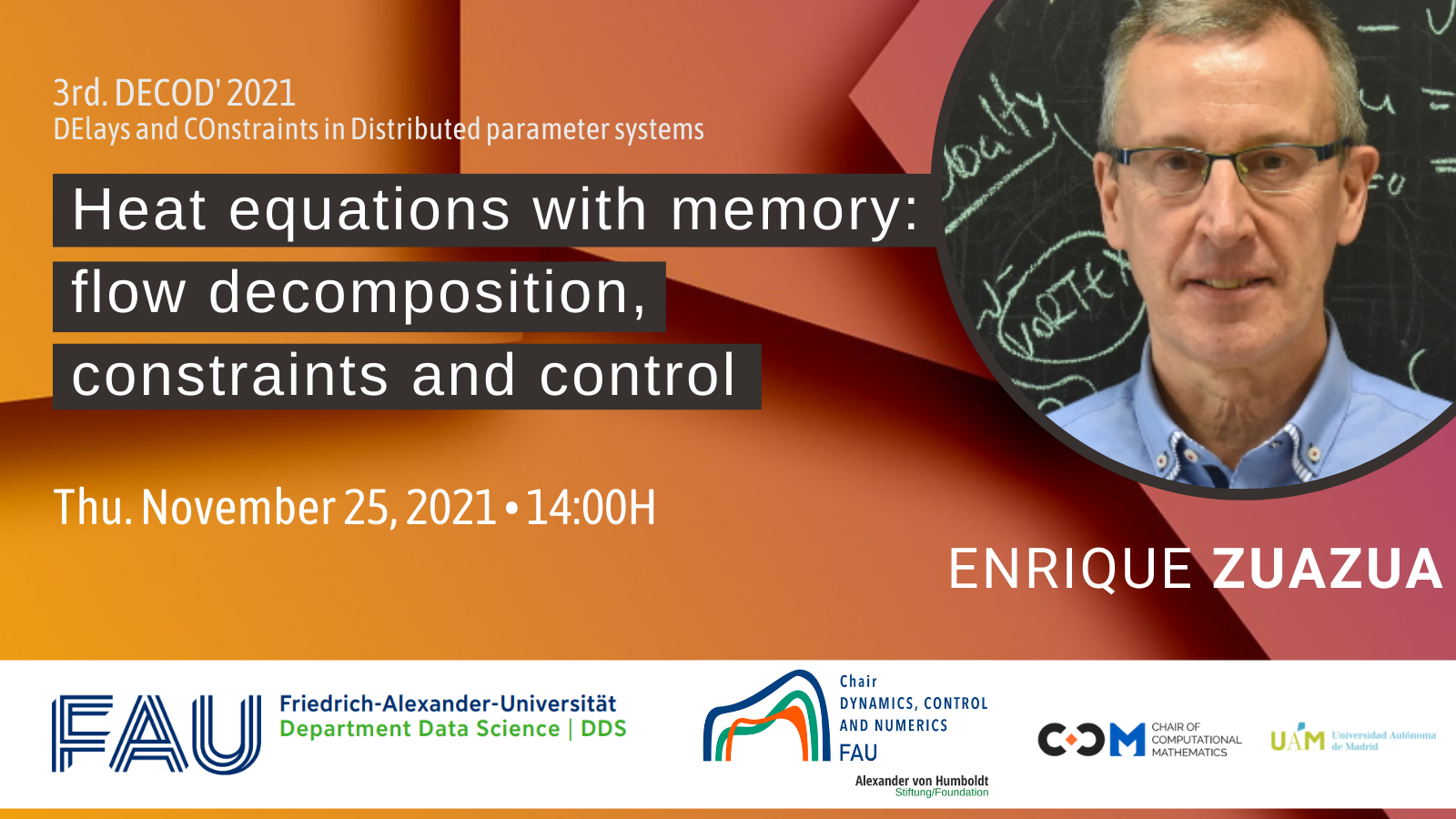# Decod2021: Heat equations with memory: flow decomposition, constraints and control by E. Zuazua

On November 25th, our Head Enrique Zuazua will give a plenary talk on Heat equations with memory: flow decomposition, constraints and control at the 3rd. DECOD — DElays and COnstraints in Distributed parameter systems- from November 23rd to 26th. at Gif-sur-Yvette (France).

Abstract. We analyze the controllability properties of heat equations involving memory terms. We interpret the system as, roughly, the coupling of a heat equation with an ordinary differential equation (ODE). The presence of the ODE for which there is no propagation along the space variable makes the controllability of the system impossible when the control is confined into a subset in space that does not move.

Following ,  and , the null controllability of the system with a moving control is established using the observability of the adjoint system and some Carleman estimates for a coupled system of a parabolic equation and an ODE with the same singular weight, adapted to the geometry of the moving support of the control. This extends to the multi-dimensional case the results of  on the one-dimensional case, employing $1-d$ Fourier analysis techniques. We also show how the techniques in  and  can be adapted to handle the same problems under pointwise constraints on states and controls. In the case of time-dependent analytic kernels, following , we also describe how the flow can be decomposed into a parabolic and a hyperbolic component, which allows to analyze from close the regularizing effect of the evolution and the propagation of singularities.

Joint work with: Felipe Chaves-Silva, Qi Lü, Lionel Rosier, Gengsheng Wang, Xu Zhang, and Yubiao Zhang.

#### WHERE?

Online (Zoom) | In presence; Yvett France: Amphi VI, Eiffel building

(Thursday, Nov 25, 2021)
Meeting ID: 998 1260 1885 | PINcode: D5ruzv

### Date

Thu. Nov 25, 2021
Expired!

14:00 - 14:45

Category